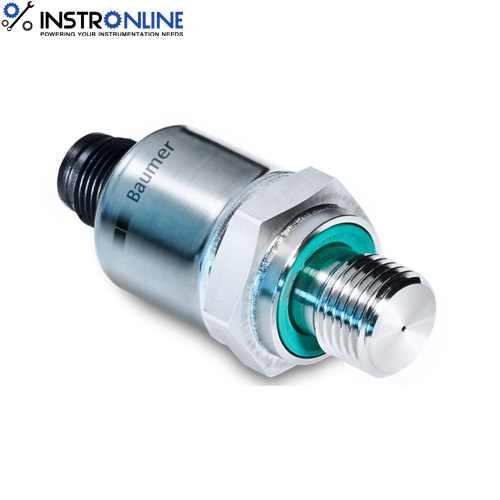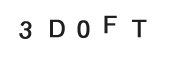• Home
• »
• Blog
• »
• Types of Sensors used in Vibration Measurement
Discussion Forum

##### Types of Sensors used in Vibration Measurement
Listed on Dec 19,2018BRIEF EXPLANATION OF VIBRATION SENSOR PRINCIPLES:

1. VELOCITY SENSORS
Electromagnetic linear velocity transducers : Typically used to measure oscillatory velocity. A permanent magnet moving back and forth within a coil winding induces an emf in the winding. This emf is proportional to the velocity of oscillation of the magnet. This permanent magnet may be attached to the vibrating object to measure its velocity.

Electromagnetic tachometer generators : Used to measure the angular velocity of vibrating objects. They provide an output voltage/frequency that is proportional to the angular velocity. DC tachometers use a permanent magnet or magneto, while the AC tachometers operate as a variable coupling transformer, with the coupling coefficient proportional to the rotary speed.

2. ACCELERATION SENSORS
Capacitive accelerometers : Used generally in those that have diaphragm supported seismic mass as a moving electrode and one/two fixed electrodes. The signal generated due to change in capacitance is post-processed using LC circuits etc., to output a measurable entity.

Piezoelectric accelerometers : Acceleration acting on a seismic mass exerts a force on the piezoelectric crystals, which then produce a proportional electric charge. The piezoelectric crystals are usually preloaded so that either an increase or decrease in acceleration causes a change in the charge produced by them. But they are not reliable at very low frequencies.

Potentiometric accelerometers : Relatively cheap and used where slowly varying acceleration is to be measured with a fair amount of accuracy. In these, the displacement of a spring mass system is mechanically linked to a viper arm, which moves along a potentiometric resistive element. Various designs may have either viscous, magnetic or gas damping.

Reluctive accelerometers : They compose accelerometers of the differential transformer type or the inductance bridge type. The AC outputs of these vary in phase as well as amplitude. They are converted into DC by means of a phase-sensitive demodulator.

Servo accelerometers : These use the closed loop servo systems of force-balance, torque-balance or null-balance to provide close accuracy. Acceleration causes a seismic mass to move. The motion is detected by one of the motion-detection devices, which generate a signal that acts as an error signal in the servo-loop. The demodulated and amplified signal is then passed through a passive damping network and then applied to the torquing coil located at the axis of rotation of the mass. The torque is proportional to the coil current, which is in turn proportional to the acceleration.

Strain Gage accelerators : these can be made very small in size and mass. The displacement of the spring-mass system is converted into a change in resistance, due to strain, in four arms of a Wheatstone bridge. The signal is then post-processed to read the acceleration.

3. PROXIMITY SENSORS
Eddy Current Sensor Probe : Eddy currents are formed when a moving (or changing) magnetic field intersects a conductor, or vice-versa. The relative motion causes a circulating flow of electrons, or currents, within the conductor. These circulating eddies of current create electromagnets with magnetic fields that oppose the effect of the applied magnetic field. The stronger the applied magnetic field, or greater the electrical conductivity of the conductor, or greater the relative velocity of motion, the greater the currents developed and the greater the opposing field Eddy current probes sense this formation of secondary fields to find out the distance between the probe and the target material.

Capacitance Proximity Sensors : Capacitive sensors use the electrical property of “capacitance” to make measurements. Capacitance is a property that exists between any two conductive surfaces within some reasonable proximity. Changes in the distance between the surfaces change the capacitance. It is this change of capacitance that capacitive sensors use to indicate changes in position of a target. High-performance displacement sensors use small sensing surfaces and as result are positioned close to the targets .Tagged Popular Searches

Related Search